Published

# Arduino Based 4-Legged Mobile Robot Built From Scratch

A 4-legged mobile robot that tracks and wiggles its tail when it sees a human face. It can be controlled from remote PC through WiFi.

IntermediateWork in progress2 days997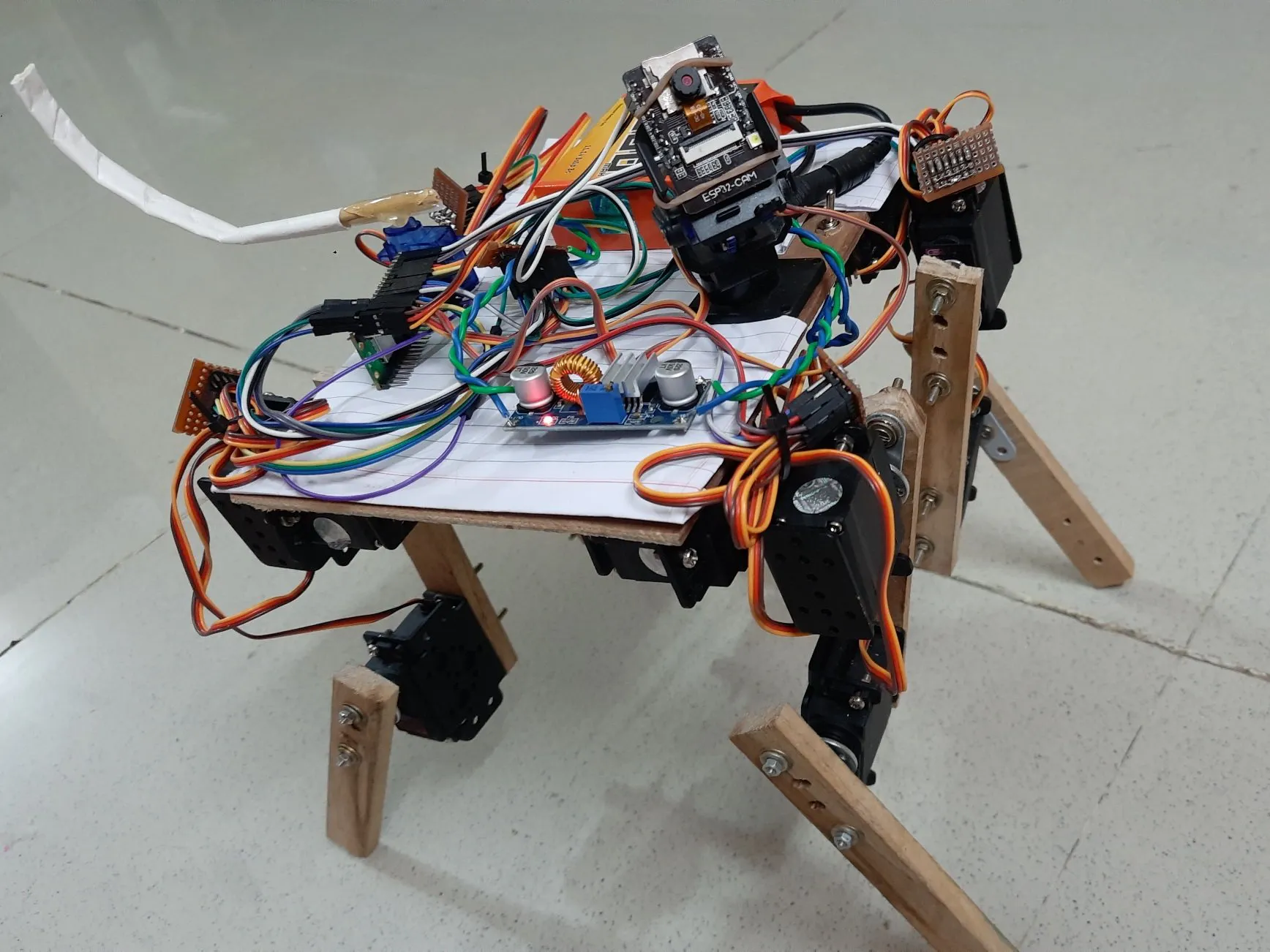## Things used in this project

### Hardware componentsArduino Nano RP2040 Connect
×1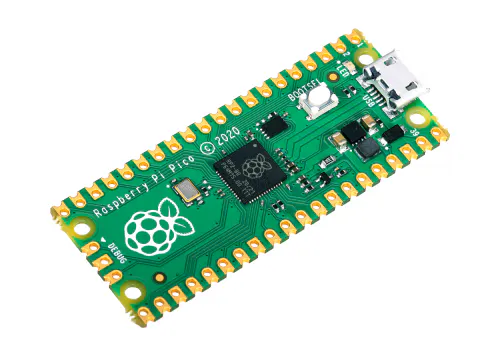Raspberry Pi Pico Actually it is Raspberry Pi Pico W
×1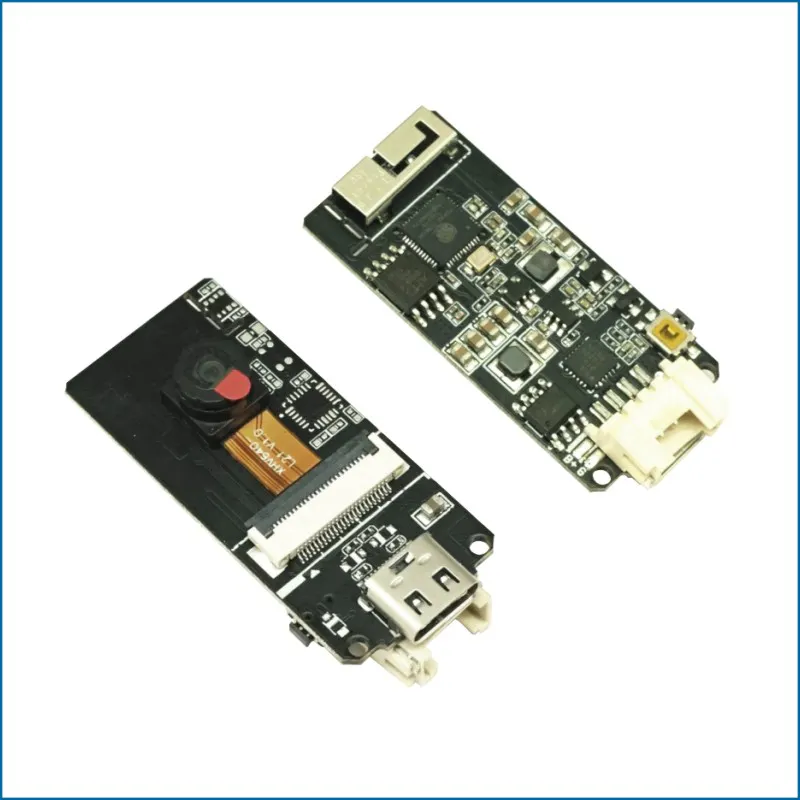M5Stack ESP32 Camera Module Development Board
×1
 12V to 6V DC Converter
×1
 High Torque Servo Motors
×12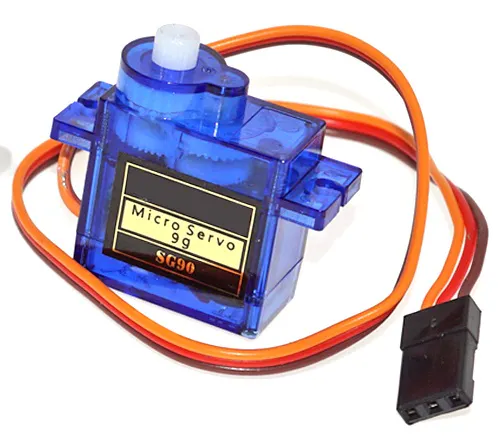DIYables Servo Motor SG90 180 degree
×1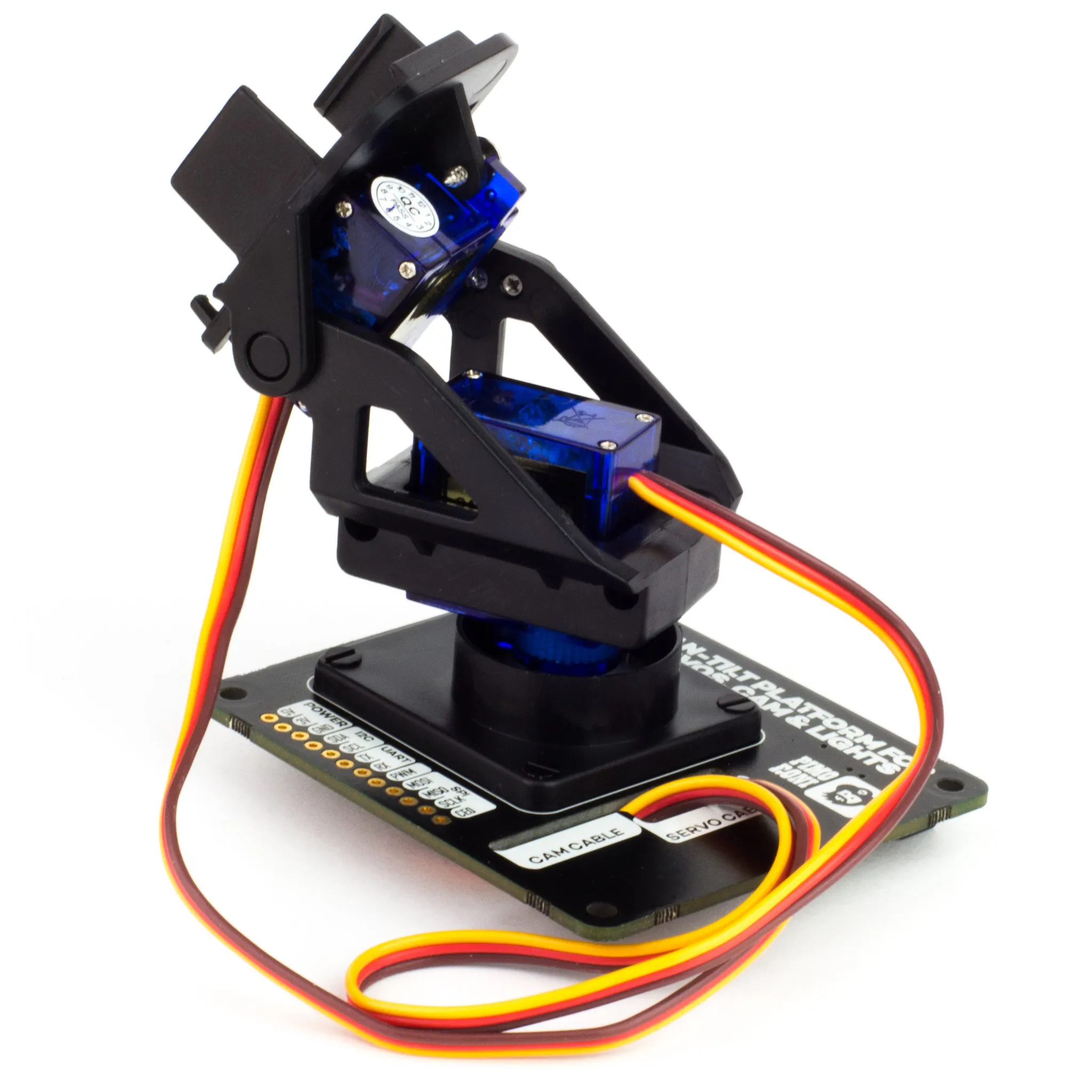Pimoroni Pan-Tilt HAT
×1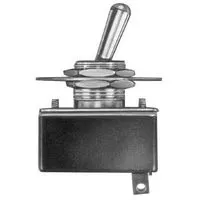Toggle Switch, Toggle
×1
 9V to 12V Battery, 2500mAH
×1
 Servo Horns
×12
 Robot mechanical componants
×1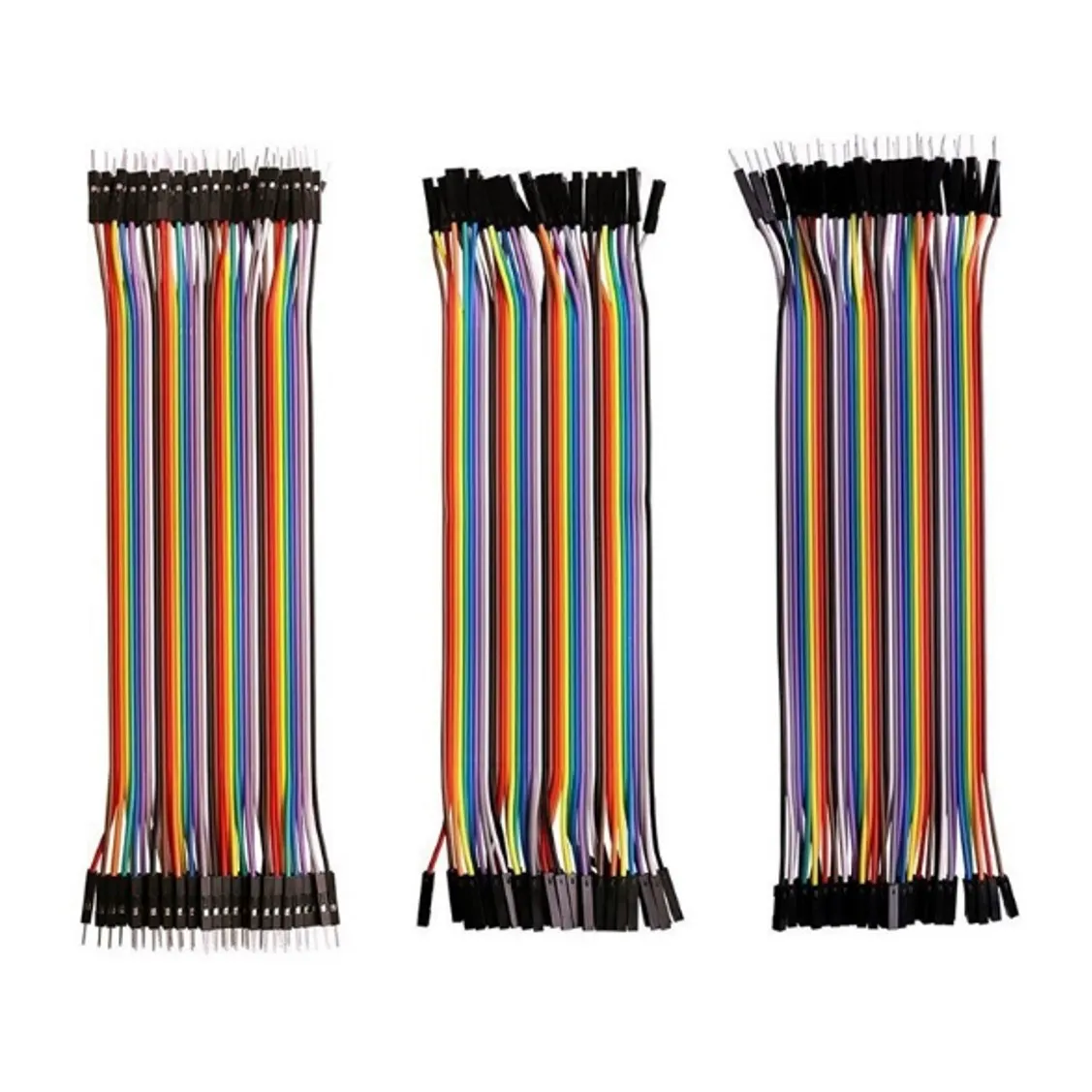DIYables Jumper Wires
×1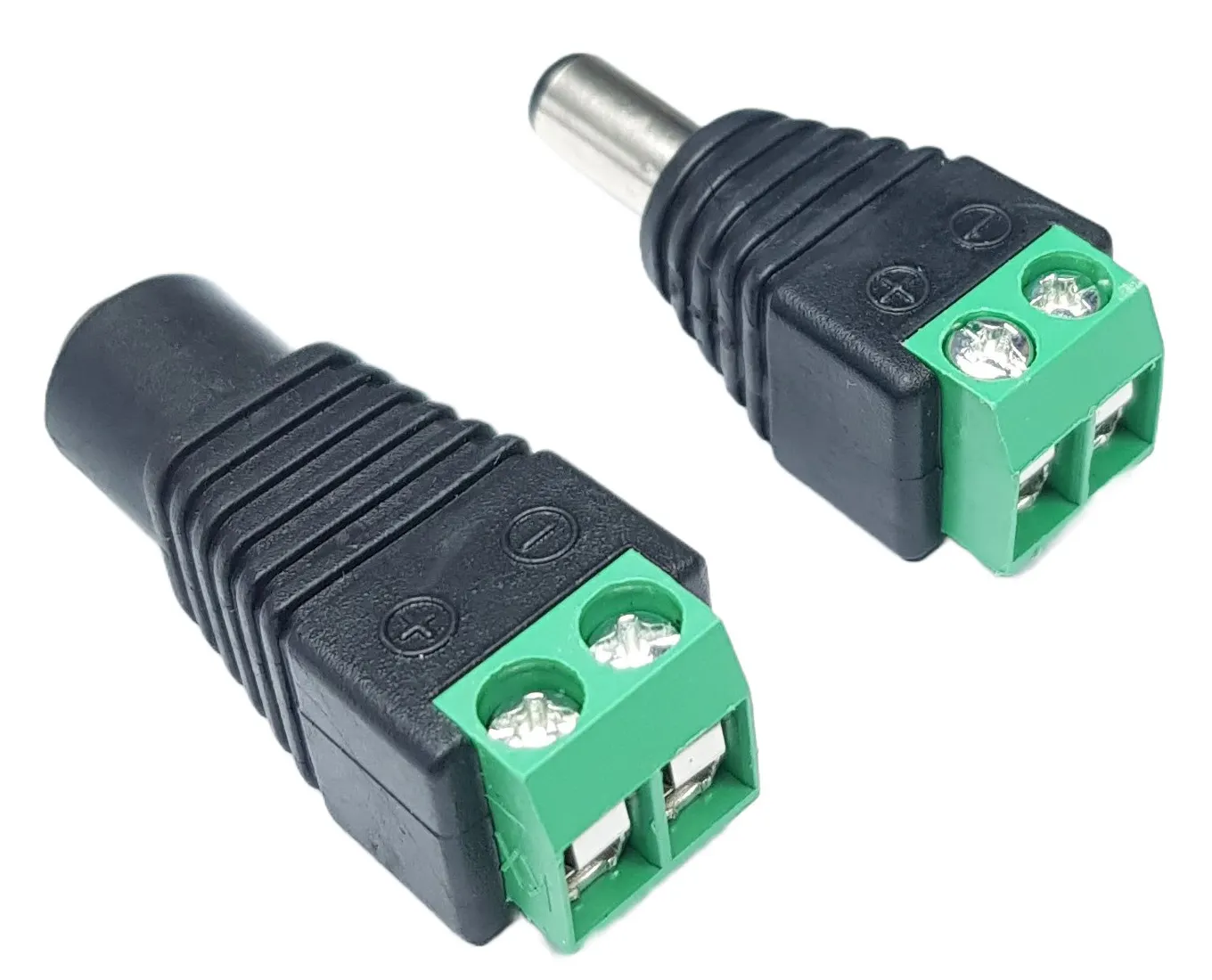DIYables DC Power Jack Connector
×1

### Software apps and online services

 Computer Aided Simulation Program (CASP)

## Schematics

### Connection Diagram for Arduino Nano RP2040 Connect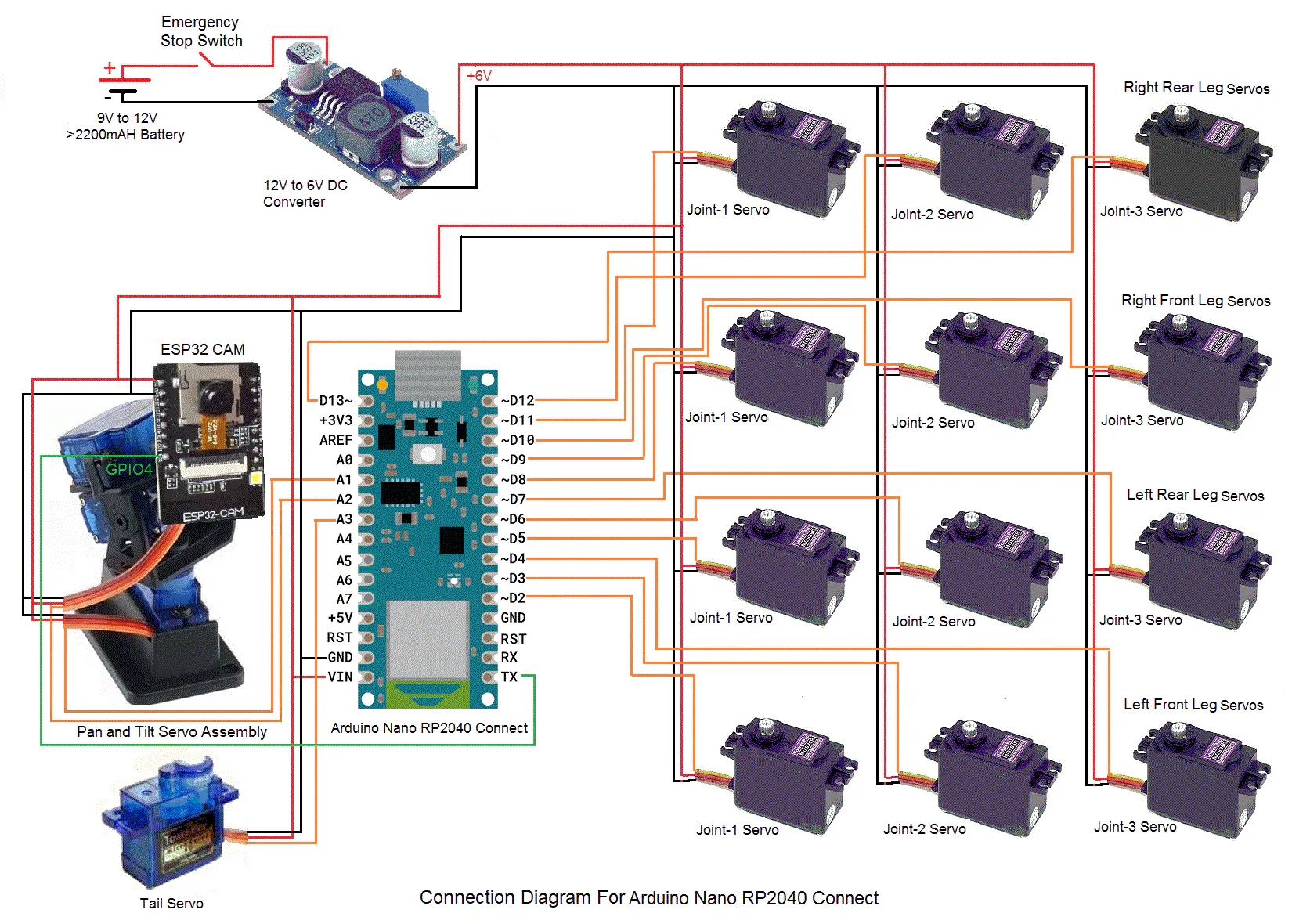### Connection Diagram for Raspberry Pi Pico W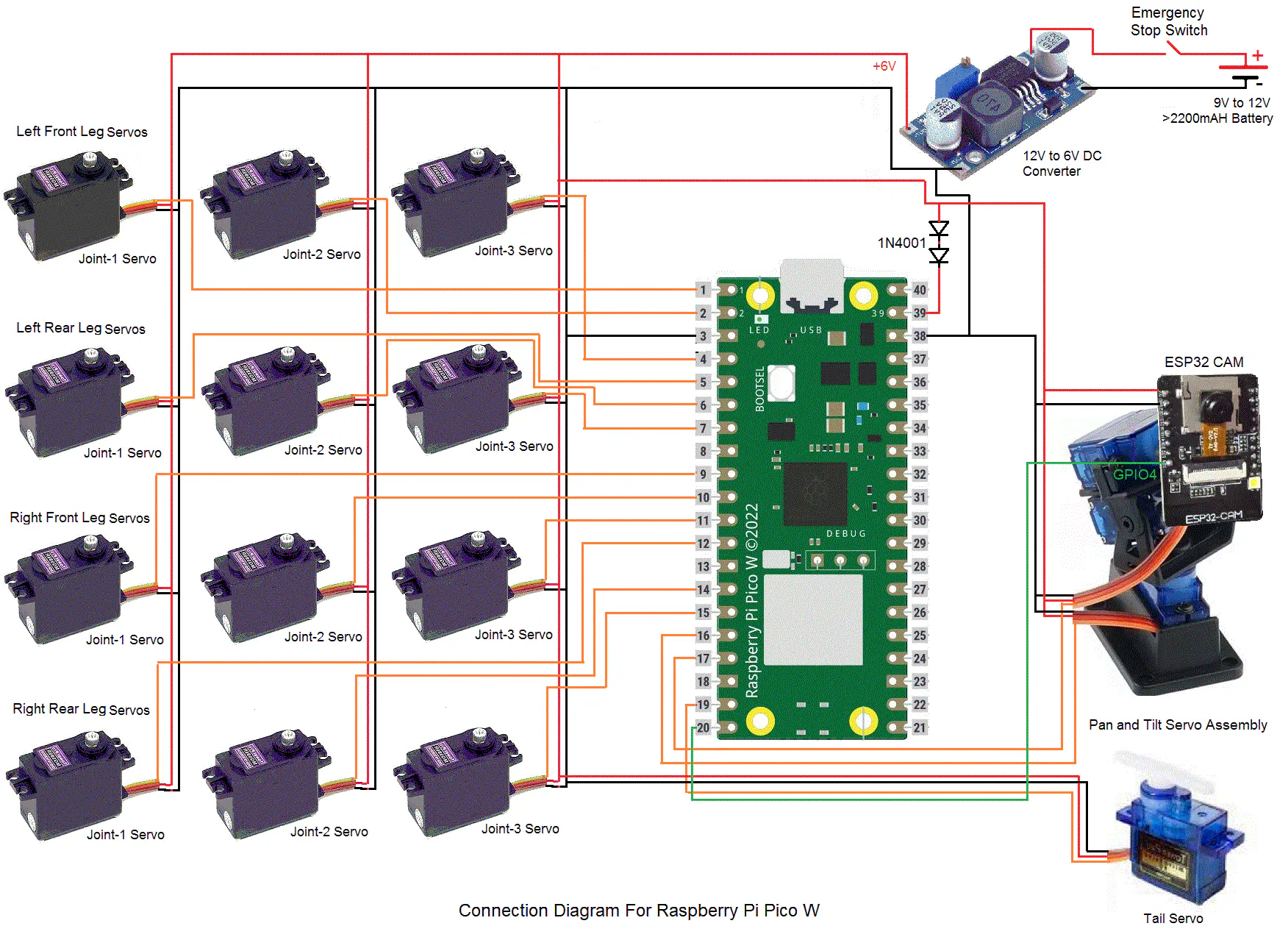### Model for Native Target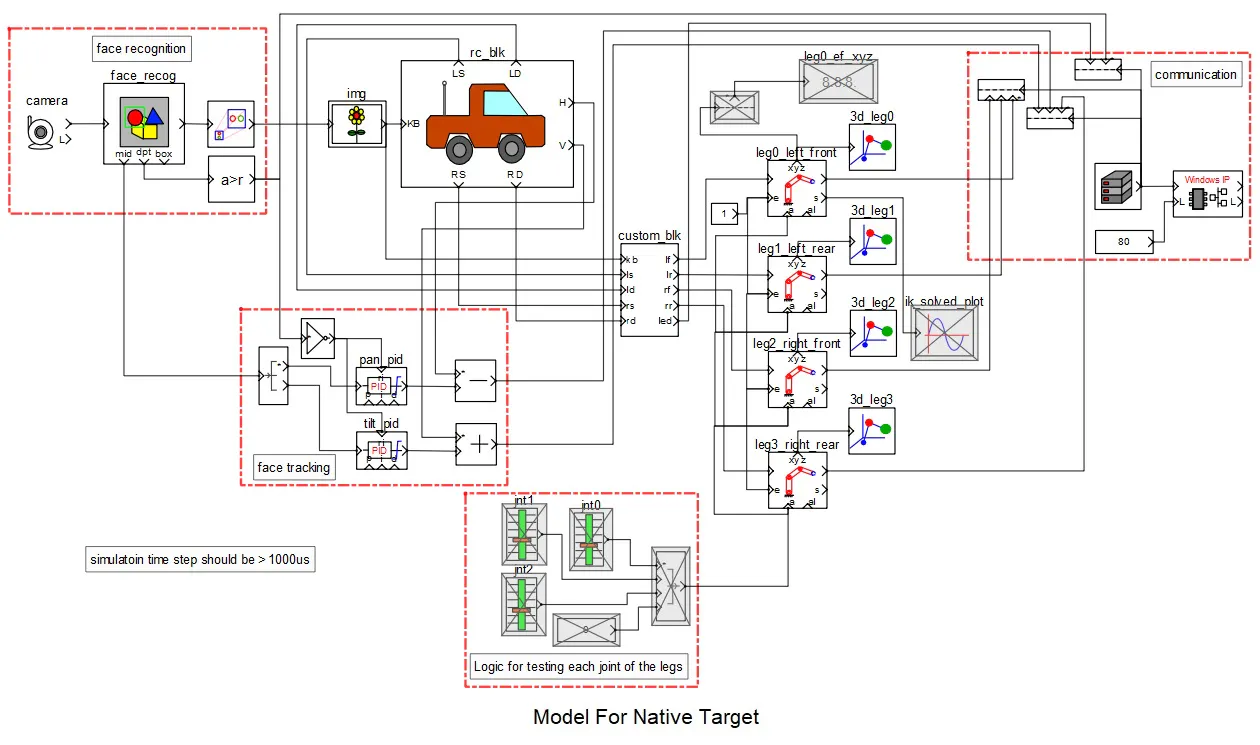## Code

### Source Code For native custom blocks

C/C++
```//CustomBlock865827210.h
#ifndef CUSTOMBLOCK865827210_H
#define CUSTOMBLOCK865827210_H

#include "sim_common_definitions.h"

//BLOCK_PREPROC_DEFS_HERE

struct LEG_STRUCT
{
int index;
XYZP offset, pt_prev;
_UINT64 time_prev;
};

#define CustomBlock865827210_LEG_AR_SIZE 36
#define CustomBlock865827210_STAND_IDX 27

= {{0,700,1}, {10,900,1.2}, {20,980,1.4}, {30,1000,1.6}, {40,950,1.8}, {50,800,2}, {60,650,2}, {70,550,2}, {80,500,2}, {90,450,2},
{100,440,2}, {110,433,2}, {120,450,2}, {130,476,2}, {140,535,2}, {150,630,1.8}, {160,800,1.6}, {170,1000,1.4}, {180,800,1.2},
{180,720,1}, {180,640,1}, {180,560,1}, {180,480,1}, {180,400,1}, {180,320,1}, {180,240,1}, {180,160,1}, {180,80,1},
{0,0,1}, {0,80,1}, {0,160,1}, {0,240,1}, {0,320,1}, {0,400,1}, {0,460,1}, {0,520,1}};

class SHARED_LIB_TYPE CustomBlock865827210
{
public:
CustomBlock865827210();
~CustomBlock865827210();
void Initialize(const volatile BLOCK_CONSTRUCTOR_PARAM_STRUCT *arg);
void PreRun(const volatile GLOBAL_PRERUN_PARAM_STRUCT *arg, _INT32 *kb_in, _FLOAT *ls_in, _INT32 *ld_in, _FLOAT *rs_in, _INT32 *rd_in, _FXDPT_FLOAT *lf_out, _FXDPT_FLOAT *lr_out, _FXDPT_FLOAT *rf_out, _FXDPT_FLOAT *rr_out, _INT32 *led_out);
void Run(const volatile GLOBAL_RUN_PARAM_STRUCT *arg, _INT32 *kb_in, _FLOAT *ls_in, _INT32 *ld_in, _FLOAT *rs_in, _INT32 *rd_in, _FXDPT_FLOAT *lf_out, _FXDPT_FLOAT *lr_out, _FXDPT_FLOAT *rf_out, _FXDPT_FLOAT *rr_out, _INT32 *led_out);
void PostRun(const volatile GLOBAL_POSTRUN_PARAM_STRUCT *arg, _INT32 *kb_in, _FLOAT *ls_in, _INT32 *ld_in, _FLOAT *rs_in, _INT32 *rd_in, _FXDPT_FLOAT *lf_out, _FXDPT_FLOAT *lr_out, _FXDPT_FLOAT *rf_out, _FXDPT_FLOAT *rr_out, _INT32 *led_out);
public:
//port variable declaration
//block internal parameter variable declaration
_FLOAT leg1_offset, leg2_offset, leg3_offset, leg4_offset;
//block internal initial condition variable declaration
private:
int GetPoint(int index, _FLOAT sp_factor, _FLOAT ang_xy, _FLOAT hmf, _FLOAT vmf, LEG_STRUCT *leg, XYZP *pt);
private:
_UINT64 m_tick;
_BYTE m_prev_led_kb;
LEG_STRUCT m_legs;//lf, rf, lr, rr
};

#endif

//CustomBlock865827210.cpp
#include "CustomBlock865827210.h"
#include "simcode.h"
#include "sim_helper.h"
#include "math.h"

//each index corresponds to 10 deg starting from 0 deg.
#define LEG_AR_SIZE CustomBlock865827210_LEG_AR_SIZE
#define LEG_AR_MAX_IDX (LEG_AR_SIZE-1)
#define LEG_AR_HALF_IDX (LEG_AR_MAX_IDX/2)
#define STAND_IDX CustomBlock865827210_STAND_IDX

CustomBlock865827210::CustomBlock865827210(){
}

CustomBlock865827210::~CustomBlock865827210(){
}

void CustomBlock865827210::Initialize(const volatile BLOCK_CONSTRUCTOR_PARAM_STRUCT *arg){
}

void CustomBlock865827210::PreRun(const volatile GLOBAL_PRERUN_PARAM_STRUCT *arg, _INT32 *kb_in, _FLOAT *ls_in, _INT32 *ld_in, _FLOAT *rs_in, _INT32 *rd_in, _FXDPT_FLOAT *lf_out, _FXDPT_FLOAT *lr_out, _FXDPT_FLOAT *rf_out, _FXDPT_FLOAT *rr_out, _INT32 *led_out){
m_tick = arg->rt_clk_tick;
for(int i=0; i<4; ++i)
{
m_legs[i].index = STAND_IDX;
m_legs[i].time_prev = arg->rt_clk_tick;
GetPoint(m_legs[i].index, -1, 0, 1, 1, &m_legs[i], 0);
}
m_legs.offset.x = leg1_offset;
m_legs.offset.y = leg1_offset;
m_legs.offset.z = leg1_offset;
//
m_legs.offset.x = leg2_offset;
m_legs.offset.y = leg2_offset;
m_legs.offset.z = leg2_offset;
//
m_legs.offset.x = leg3_offset;
m_legs.offset.y = leg3_offset;
m_legs.offset.z = leg3_offset;
//
m_legs.offset.x = leg4_offset;
m_legs.offset.y = leg4_offset;
m_legs.offset.z = leg4_offset;
//
base_speed = base_speed/1000;//convert to mm/msec
m_prev_led_kb = 0;
}

void CustomBlock865827210::Run(const volatile GLOBAL_RUN_PARAM_STRUCT *arg, _INT32 *kb_in, _FLOAT *ls_in, _INT32 *ld_in, _FLOAT *rs_in, _INT32 *rd_in, _FXDPT_FLOAT *lf_out, _FXDPT_FLOAT *lr_out, _FXDPT_FLOAT *rf_out, _FXDPT_FLOAT *rr_out, _INT32 *led_out){
//if(arg->rt_clk_tick-m_tick < 1000)
//    return;
m_tick = arg->rt_clk_tick;
//flash light led logic
if(kb_in)//L
{
if(m_prev_led_kb == 0)
{
m_prev_led_kb = 1;
*led_out = (*led_out)?0:1;
}
}
else
m_prev_led_kb = 0;
//
_FLOAT ls = *ls_in;
_FLOAT rs = *rs_in;
_FLOAT sp = (ls + rs)/2;//average speed
int ldir = *ld_in;//1-forward, 0-backward
int rdir = (*rd_in)?0:1;
if(sp < 0.001)//standing
{
sp = 1.0;
m_legs.index = m_legs.index = m_legs.index = m_legs.index = STAND_IDX;
GetPoint(m_legs.index, sp, 0, 1, 1, &m_legs, (XYZP*)lf_out);
GetPoint(m_legs.index, sp, 0, 1, 1, &m_legs, (XYZP*)lr_out);
GetPoint(m_legs.index, sp, 0, 1, 1, &m_legs, (XYZP*)rf_out);
GetPoint(m_legs.index, sp, 0, 1, 1, &m_legs, (XYZP*)rr_out);
}
else//walk
{
int leg_mag_idx;
_FLOAT leg_ang;
_FLOAT hmul, vmul;
//
leg_mag_idx = m_legs.index+0;//left front leg
leg_mag_idx = m_legs.index+LEG_AR_HALF_IDX;//left rear leg
leg_mag_idx = m_legs.index+LEG_AR_HALF_IDX;//right front leg
leg_mag_idx = m_legs.index+0;//right rear leg
//
if(ldir == 1 && rdir == 0)//rotate right
{
if(kb_in)
{
leg_ang = leg_ang = 90;
leg_ang = leg_ang = 90;
}
else
{
leg_ang = leg_ang = 90;
leg_ang = leg_ang = -90;
}
//
hmul = 0.5;
vmul = 1.0;
sp *= 1.2;
}
else if(ldir == 0 && rdir == 1)//rotate left
{
if(kb_in)
{
leg_ang = leg_ang = -90;
leg_ang = leg_ang = -90;
}
else
{
leg_ang = leg_ang = -90;
leg_ang = leg_ang = 90;
}
//
hmul = 0.5;
vmul = 1.0;
sp *= 1.2;
}
else if(ldir == 1 && rdir == 1)//move forward
{
if(IsEqual(ls, rs))
{
leg_ang = leg_ang = leg_ang = leg_ang = 0;
}
else if(ls > rs)//go right
{
leg_ang = leg_ang = 30;
leg_ang = leg_ang = -30;
}
else//ls < rs, go left
{
leg_ang = leg_ang = -30;
leg_ang = leg_ang = 30;
}
hmul = vmul = 1.0;
}
else//move backward
{
if(IsEqual(ls, rs))
{
leg_ang = leg_ang = leg_ang = leg_ang = 180;
}
else if(ls > rs)//go right
{
leg_ang = leg_ang = (180+30);
leg_ang = leg_ang = (180-30);
}
else//ls < rs, go left
{
leg_ang = leg_ang = (180-30);
leg_ang = leg_ang = (180+30);
}
hmul = vmul = 1.0;
}
//get current x,y,z co-ordinates for all 4 legs
int ret = GetPoint(leg_mag_idx, sp, leg_ang, hmul, vmul, &m_legs, (XYZP*)lf_out);
m_legs.index += (ret && (m_legs.index<LEG_AR_MAX_IDX))?1:0;
//
ret = GetPoint(leg_mag_idx, sp, leg_ang, hmul, vmul, &m_legs, (XYZP*)lr_out);
m_legs.index += (ret && (m_legs.index<LEG_AR_MAX_IDX))?1:0;
//
ret = GetPoint(leg_mag_idx, sp, leg_ang, hmul, vmul, &m_legs, (XYZP*)rf_out);
m_legs.index += (ret && (m_legs.index<LEG_AR_MAX_IDX))?1:0;
//
ret = GetPoint(leg_mag_idx, sp, leg_ang, hmul, vmul, &m_legs, (XYZP*)rr_out);
m_legs.index += (ret && (m_legs.index<LEG_AR_MAX_IDX))?1:0;
//synchronise all four legs
if(m_legs.index == LEG_AR_MAX_IDX &&  m_legs.index == LEG_AR_MAX_IDX && m_legs.index == LEG_AR_MAX_IDX && m_legs.index == LEG_AR_MAX_IDX)
{
m_legs.index = 0;
m_legs.index = 0;
m_legs.index = 0;
m_legs.index = 0;
}
}
}

void CustomBlock865827210::PostRun(const volatile GLOBAL_POSTRUN_PARAM_STRUCT *arg, _INT32 *kb_in, _FLOAT *ls_in, _INT32 *ld_in, _FLOAT *rs_in, _INT32 *rd_in, _FXDPT_FLOAT *lf_out, _FXDPT_FLOAT *lr_out, _FXDPT_FLOAT *rf_out, _FXDPT_FLOAT *rr_out, _INT32 *led_out){
//standing
_FLOAT sp = 1.0;
m_legs.index = m_legs.index = m_legs.index = m_legs.index = STAND_IDX;
GetPoint(m_legs.index, sp, 0, 1, 1, &m_legs, (XYZP*)lf_out);
GetPoint(m_legs.index, sp, 0, 1, 1, &m_legs, (XYZP*)lr_out);
GetPoint(m_legs.index, sp, 0, 1, 1, &m_legs, (XYZP*)rf_out);
GetPoint(m_legs.index, sp, 0, 1, 1, &m_legs, (XYZP*)rr_out);
}

int CustomBlock865827210::GetPoint(int index, _FLOAT sp_factor, _FLOAT ang_xy, _FLOAT hmf, _FLOAT vmf, LEG_STRUCT *leg, XYZP *pt)
{
if(IsZero(sp_factor))
{
leg->time_prev = m_tick;
return 1;
}
//check limits
if(index < 0) index = LEG_AR_SIZE + index;
if(index > LEG_AR_MAX_IDX) index = index-LEG_AR_SIZE;
//
_FLOAT ang = g_CustomBlock865827210_leg_mag[index];//angle
_FLOAT speed = g_CustomBlock865827210_leg_mag[index];//speed
XYZP p;
p.x = 0;
//convert to user angle
ang = ang_xy;
p.x = rad * sin(ang * MATH_DEG_TO_RAD);//sin because we r taking along y as angle zero
//adjust point wrt speed. only if sp_factor > 0
int ret = 1;
if(sp_factor > 0.0001)
{
_FLOAT len = GetDistance(&p, &leg->pt_prev);
if(len > 0.001)
{
_FLOAT td = (m_tick-leg->time_prev)/1000.0;//in msec
_FLOAT dis = td * (base_speed*sp_factor*speed);
_FXDPT_FLOAT ratio = dis/len;
if(ratio > 1) ratio = 1;
p.x = leg->pt_prev.x + ((p.x-leg->pt_prev.x)*ratio);
p.y = leg->pt_prev.y + ((p.y-leg->pt_prev.y)*ratio);
p.z = leg->pt_prev.z + ((p.z-leg->pt_prev.z)*ratio);
ret = 0;
}
}
leg->time_prev = m_tick;
leg->pt_prev.x = p.x;
leg->pt_prev.y = p.y;
leg->pt_prev.z = p.z;
if(pt)
{
//translate
p.x = p.x + leg->offset.x;
p.y = p.y + leg->offset.y;
p.z = p.z + leg->offset.z;
//
pt->x = p.x;
pt->y = p.y;
pt->z = p.z;
}
return ret;
}
```